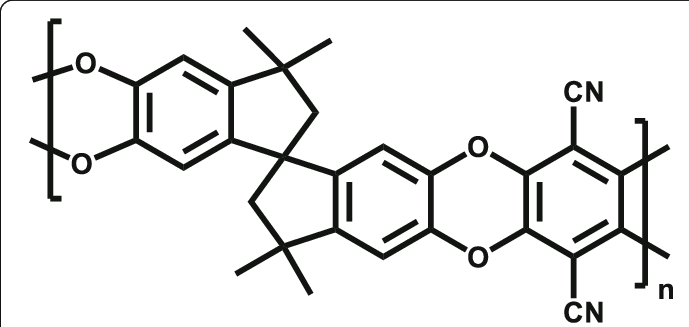# Can you build ladder polymers using EMC?

Hi!

I am a fairly new EMC user and have been using the package to set up all-atom polymer systems for simulation in LAMMPS using the PCFF force field. I’ve been extremely impressed so far with how robust EMC is and how easy it is to generate different molecules and polymer morphologies by entering only a SMILES string and specifying the connectivity points.

Some of the systems that I’m interested in simulating are so-called “ladder polymers” like PIM-1. For these molecules, each monomer has four connection points instead of two, and when two monomers are joined they are connected by two separate ether linkages that join rings together into a planar structure.My question is this: is there a way to build these kinds of polymers directly in EMC? I haven’t been able to figure one out directly, and I’m not sure if it’s because this is a feature that isn’t implemented or just because of my unfamiliarity. For what it’s worth my current solution is to build polymer chains that are connected by only one of the links and then attempt to close the second one using reactive MD in LAMMPS.

Thank you so much!

Sam

Hi Sam,

Thank you for your question. Your polymer appears to me to be a linear polymer, which – with a little bit of reorganizing – more of less abides by the following SMILES:

``````CC1(C)CC(*)C2=CC3=C(OC4=C(C#N)C5=C(OC6=CC7=C(C=C6O5)C(*)CC7(C)C)C(C#N)=C4O3)C=C12
``````

Unfortunately, the latter does not quite have the repeat unit connectivity you need. In essence, two adjacent repeat units share a carbon in a saturated five ring an do not connect between carbons in two separate saturated five rings. A SMILES reflecting this connectivity could look like

``````CC1(C)C[*C]C2=CC3=C(OC4=C(C#N)C5=C(OC6=CC7=C(C=C6O5)[*C]CC7(C)C)C(C#N)=C4O3)C=C12
``````

where `[*C]` reflect the shared carbon in both five rings. Unfortunately, this SMILES representation is currently not supported by EMC. In principle, the building mechanism should be able to handle the polymer though, if the representation could be interpreted.

The monomer unit itself is very stiff. This can be seen when building the monomer itself with e.g. fluor at the connection points:

``````CC1(C)CC(F)C2=CC3=C(OC4=C(C#N)C5=C(OC6=CC7=C(C=C6O5)C(F)CC7(C)C)C(C#N)=C4O3)C=C12
``````

This de facto means, that packing the molecule during build might be a challenge as well. A polymer consisting of this monomer displays a rather rigid zigzag structure, which does not help either.

1 Like

Hi Pieter,